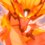# Generalized Harmonic Summation

Evaluate

$\large{\sum_{r=1}^{\infty} \dfrac{H_{r} ^{(m)}}{r^m}} \quad ; \quad m \geq 2$

Notation: $H_{r} ^{(m)}$ denotes the Generalized Harmonic Number.

This is a part of the set Formidable Series and IntegralsNote by Ishan Singh
3 years, 8 months ago

This discussion board is a place to discuss our Daily Challenges and the math and science related to those challenges. Explanations are more than just a solution — they should explain the steps and thinking strategies that you used to obtain the solution. Comments should further the discussion of math and science.

When posting on Brilliant:

• Use the emojis to react to an explanation, whether you're congratulating a job well done , or just really confused .
• Ask specific questions about the challenge or the steps in somebody's explanation. Well-posed questions can add a lot to the discussion, but posting "I don't understand!" doesn't help anyone.
• Try to contribute something new to the discussion, whether it is an extension, generalization or other idea related to the challenge.

MarkdownAppears as
*italics* or _italics_ italics
**bold** or __bold__ bold
- bulleted- list
• bulleted
• list
1. numbered2. list
1. numbered
2. list
Note: you must add a full line of space before and after lists for them to show up correctly
paragraph 1paragraph 2

paragraph 1

paragraph 2

[example link](https://brilliant.org)example link
> This is a quote
This is a quote
    # I indented these lines
# 4 spaces, and now they show
# up as a code block.

print "hello world"
# I indented these lines
# 4 spaces, and now they show
# up as a code block.

print "hello world"
MathAppears as
Remember to wrap math in $$ ... $$ or $ ... $ to ensure proper formatting.
2 \times 3 $2 \times 3$
2^{34} $2^{34}$
a_{i-1} $a_{i-1}$
\frac{2}{3} $\frac{2}{3}$
\sqrt{2} $\sqrt{2}$
\sum_{i=1}^3 $\sum_{i=1}^3$
\sin \theta $\sin \theta$
\boxed{123} $\boxed{123}$

Sort by:

I have solved it by $4$ methods. One is Summation By Parts, other are the following :

First, expand the summation, using the definition of $\displaystyle H_{r}^{(m)}$ , to see that $\displaystyle \sum_{r=1}^{n} \dfrac{H_{r}^{(m)}}{r^m} = \sum_{r=1}^{n} \dfrac{1}{r^{2m}} + \sum_{r < j} \sum_{j=2}^{n} \dfrac{1}{rj} = H_{n}^{(2m)} + \sum_{r < j} \sum_{j=2}^{n} \dfrac{1}{rj}$

Also,

$\displaystyle [H_{n}^{(m)}]^2 = H_{n}^{(2m)} + 2\sum_{r < j} \sum_{j=2}^{n} \dfrac{1}{rj}$

Eliminating $\displaystyle \sum_{r < j} \sum_{j=2}^{n} \dfrac{1}{rj}$ from the above equations, we have,

$\displaystyle \sum_{r=1}^{n} \dfrac{H_{r}^{(m)}}{r^m} =\dfrac{1}{2} \left( [H_{n}^{(m)}]^2 + H_{n}^{(2m)} \right) \tag{*}$

Now take limit to infinity to get the desired result.

Also note that $(*)$ holds for $m=1$ as well (but the infinite sum diverges).

My other two methods use integral representations of $\displaystyle H_{r}^{(m)}$ and $\dfrac{1}{r^m}$. For instance, I have used one of them here.

- 3 years, 8 months ago

Yes even I thought about the same method! Shuffling indeed helps.

- 3 years, 8 months ago

$\displaystyle S=\lim _{ n\rightarrow \infty }{ \sum _{ r=1 }^{ n }{ \frac { { H }_{ r }^{ \left( m \right) } }{ { r }^{ m } } } }$

By Summation by parts, we get:

$\displaystyle S=\lim _{ n\rightarrow \infty }{ { H }_{ n }^{ \left( m \right) }{ H }_{ n+1 }^{ \left( m \right) }-\sum _{ r=1 }^{ n }{ { H }_{ r }^{ \left( m \right) }\left( { H }_{ r+1 }^{ \left( m \right) }-{ H }_{ r }^{ \left( m \right) } \right) } }$

Now we use ${ H }_{ r+1 }^{ \left( m \right) }={ H }_{ r }^{ \left( m \right) }+\frac { 1 }{ { \left( r+1 \right) }^{ m } }$

$\displaystyle S=\lim _{ n\rightarrow \infty }{ { H }_{ n }^{ \left( m \right) }{ H }_{ n+1 }^{ \left( m \right) }-\sum _{ r=1 }^{ n }{ \frac { { H }_{ r }^{ \left( m \right) } }{ { \left( r+1 \right) }^{ m } } } }$

Again, we use ${ H }_{ r+1 }^{ \left( m \right) }={ H }_{ r }^{ \left( m \right) }+\frac { 1 }{ { \left( r+1 \right) }^{ m } }$

$\displaystyle S=\lim _{ n\rightarrow \infty }{ { H }_{ n }^{ \left( m \right) }{ H }_{ n+1 }^{ \left( m \right) }-S+1+\sum _{ r=1 }^{ n }{ \frac { 1 }{ { \left( r+1 \right) }^{ 2m } } } }$

On simplifying, we get:

$\boxed{S=\frac { { \zeta }^{ 2 }\left( m \right) +\zeta \left( 2m \right) }{ 2 } }$

- 3 years, 8 months ago

What do you mean by 'summation by parts'?

- 3 years, 8 months ago

It is analogous to "Integration By Parts".

- 3 years, 8 months ago

Please correct me if I'm wrong.

- 3 years, 8 months ago

(+1) Correct!

- 3 years, 8 months ago

- 3 years, 8 months ago

Nice note!

- 3 years, 8 months ago

I now one value of this.

Which is the first one.

- 3 years, 8 months ago